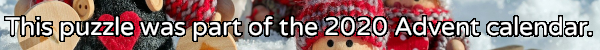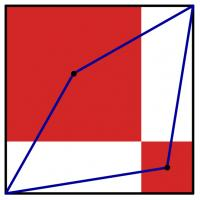mscroggs.co.uk
mscroggs.co.uksubscribe

# Puzzles

## 17 DecemberPut the digits 1 to 9 (using each digit exactly once) in the boxes so that the sums and product are correct. Today's number is the product of the numbers in the red boxes.
 + + = 16 + + + + + = 8 + + + × × = 288 =11 =14 =20
Tags: numbers, grids

## 16 DecemberSolve the crossnumber to find today's number. No number starts with 0.

## 15 DecemberWhen talking to someone about this Advent calendar, you told them that the combination of XMAS and MATHS is GREAT. They were American, so asked you if the combination of XMAS and MATH is great; you said SURE. You asked them their name; they said SAM.
Each of the letters E, X, M, A, T, H, S, R, U, and G stands for a different digit 0 to 9. The following sums are correct:
Today's number is SAM. To help you get started, the letter T represents 4.

## 14 DecemberThe numbers 33, 404 and 311 contain duplicate digits. The numbers 120, 15 and 312 do not.
How many numbers between 10 and 999 (inclusive) contain no duplicate digits?

## 13 DecemberThere are 6 ways to split the sequence of the numbers 1 to 5 into three shorter sequences:
• 1 and 2 and 3, 4, 5
• 1 and 2, 3 and 4, 5
• 1 and 2, 3, 4 and 5
• 1, 2 and 3 and 4, 5
• 1, 2 and 3, 4 and 5
• 1, 2, 3 and 4 and 5
Today's number is the number of ways to split the sequence of the numbers 1 to 10 into five shorter sequences.

## 12 DecemberThe diagram to the left shows a large black square. Inside this square, two red squares have been drawn. (The sides of the red squares are parallel to the sides of the black square; each red square shares a vertex with the black square; and the two red squares share a vertex.) A blue quadrilateral has then been drawn with vertices at two corners of the black square and the centres of the red squares.
The area of the blue quadrilateral is 167. What is the area of the black square?

## 11 DecemberNoel has a large pile of cards. Half of them are red, the other half are black. Noel splits the cards into two piles: pile A and pile B.
Two thirds of the cards in pile A are red. Noel then moves 108 red cards from pile A to pile B. After this move, two thirds of the cards in pile B are red.